﻿ 旋转检偏技术的理论分析及其在电光效应实验中应用 Theoretical Analysis of Rotating Polarization-Analyzer for Polarized Light and Its Application in Photoelectric Effect Experiment

Modern Physics
Vol.08 No.01(2018), Article ID:23855,10 pages
10.12677/MP.2018.81002

Theoretical Analysis of Rotating Polarization-Analyzer for Polarized Light and Its Application in Photoelectric Effect Experiment

Dangbo Liu1,2*, Changchun Ning3*, Haixiang Gao1, Yifan Gao1, Chunming Gu1

1Department of Astronomy, School of Physical and Astronomy, Shangha Jiao Tong University, Shanghai

2Shanghai Key Laboratory for Particle Physics and Cosmology, Shanghai Jiao Tong University, Shanghai

3Department of Physics, College of Science, Tibet University, Lhasa Tibet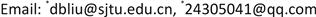Received: Feb. 6th, 2018; accepted: Feb. 20th, 2018; published: Feb. 27th, 2018ABSTRACT

We developed innovative polarization analyzer and used it in the photoelectric effect experiment. We give a detailed analysis of innovative rotating polarization analyzer, and calculate the distribution curves of the emergent intensity of emitting light. The analyzer was rotated by a mini-motor and polarization beam was returned to electric signal then to a computer by a photoelectric behind it. A BBO crystal was used and its 1/4 wave voltage is about 1200 V. The experimental and simulated results are in good agreement with each other and show that this method is effective. A key point is that we can determine the orientation of the light axis of a crystal.

Keywords:Rotating Polarization Analyzer, Marius's Law, Interference of Polarized Light, 8 Shape of Polarized Light

1上海交通大学 物理与天文学院 天文系，上海

2上海交通大学 上海粒子物理和宇宙学重点实验室，上海

3西藏大学 理学院 物理系，西藏 拉萨1. 引言

2. 旋转检偏理论简介

2.1. 椭圆偏振光的起偏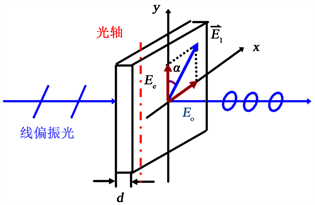Figure 1. The polarization of elliptically (circularly) polarized light

${E}_{o}={E}_{1}\mathrm{sin}\alpha ,\text{ }{E}_{e}={E}_{1}\mathrm{cos}\alpha \text{\hspace{0.17em}}.$

$\delta =\frac{2\text{π}}{\lambda }\left({n}_{o}-{n}_{e}\right)d$ (1)

${E}_{x}={E}_{o}\mathrm{cos}\omega t\text{\hspace{0.17em}},\text{ }{E}_{y}={E}_{e}\mathrm{cos}\left(\omega t+\delta \right)$

$\frac{{E}_{x}^{2}}{{E}_{o}^{2}}+\frac{{E}_{y}^{2}}{{E}_{e}^{2}}-\frac{2{E}_{x}{E}_{y}}{{E}_{o}{E}_{e}}\mathrm{cos}\delta ={\mathrm{sin}}^{2}\delta$ (2)

2.2. 偏振光的干涉及旋转检偏

${E}_{o2}=\left\{\begin{array}{l}{E}_{o}\mathrm{sin}\left(\beta -\alpha \right)={E}_{1}\mathrm{sin}\alpha \mathrm{sin}\left(\beta -\alpha \right),\text{ }\text{ }\text{ }\text{\hspace{0.17em}}\text{ }0<\beta -\alpha <\text{π}/\text{2},\\ {E}_{o}\mathrm{sin}\left[\text{π}-\left(\beta -\alpha \right)\right]={E}_{1}\mathrm{sin}\alpha \mathrm{sin}\left(\beta -\alpha \right),\text{ }\text{π}/\text{2}<\beta -\alpha <\text{π}\text{ }\text{.}\end{array}$ (3)

${E}_{e2}=\left\{\begin{array}{l}{E}_{e}\mathrm{cos}\left(\beta -\alpha \right)={E}_{1}\mathrm{cos}\alpha \mathrm{cos}\left(\beta -\alpha \right),\text{\hspace{0.17em}}\text{ }\text{ }\text{ }\text{\hspace{0.17em}}\text{\hspace{0.17em}}\text{ }0<\beta -\alpha <\text{π}/\text{2},\\ {E}_{e}\mathrm{cos}\left[\text{π}-\left(\beta -\alpha \right)\right]=-{E}_{1}\mathrm{cos}\alpha \mathrm{cos}\left(\beta -\alpha \right),\text{ }\text{π}/\text{2}<\beta -\alpha <\text{π}\text{ }\text{.}\end{array}$ (4)

${E}_{\text{2}}={E}_{o2}+{E}_{e2}\text{\hspace{0.17em}}.$ (5)

${\delta }_{出}={\delta }_{入}+\delta +{\delta }_{投}$ (6)

${E}_{2}=\sqrt{{E}_{o2}^{2}+{E}_{e2}^{2}+2{E}_{o2}{E}_{e2}\mathrm{cos}{\delta }_{出}}$ (7)

${\delta }_{出}={\delta }_{入}+\delta +{\delta }_{投}=\frac{2\text{π}}{\lambda }\left({n}_{o}-{n}_{e}\right)d+\left\{\begin{array}{l}\text{π},\text{\hspace{0.17em}}\text{ }\text{\hspace{0.17em}}0<\beta -\alpha <\text{π}/2,\\ 0\text{\hspace{0.17em}}\text{\hspace{0.17em}},\text{ }\text{ }\text{π}/\text{2}<\beta -\alpha <\text{π}\text{ }\text{.}\end{array}$ (8)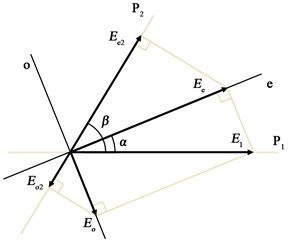(a)(b)

Figure 2. Interference of the polarized light; The resolution and composition of the electric field vector

$\begin{array}{c}\frac{{I}_{2}}{{I}_{1}}=\frac{{E}_{2}^{2}}{{E}_{1}^{2}}=\frac{{E}_{o2}^{2}+{E}_{e2}^{2}+2{E}_{o2}{E}_{e2}\mathrm{cos}{\delta }_{出}}{{E}_{1}^{2}}\\ ={\mathrm{sin}}^{2}\alpha {\mathrm{sin}}^{2}\left(\beta -\alpha \right)+{\mathrm{cos}}^{2}\alpha {\mathrm{cos}}^{2}\left(\beta -\alpha \right)\\ \text{\hspace{0.17em}}\text{\hspace{0.17em}}-2\mathrm{sin}\alpha \mathrm{sin}\left(\beta -\alpha \right)\mathrm{cos}\alpha \mathrm{cos}\left(\beta -\alpha \right)\mathrm{cos}\delta \\ ={\mathrm{cos}}^{2}\left(\beta -2\alpha \right)-\mathrm{sin}2\alpha \mathrm{sin}\left(2\beta -2\alpha \right){\mathrm{cos}}^{2}\left(\delta /2\right)\\ ={\mathrm{cos}}^{2}\beta +\mathrm{sin}2\alpha \mathrm{sin}\left(2\beta -2\alpha \right){\mathrm{sin}}^{2}\left(\delta /2\right)\end{array}$ (9)

3. 实验设计思路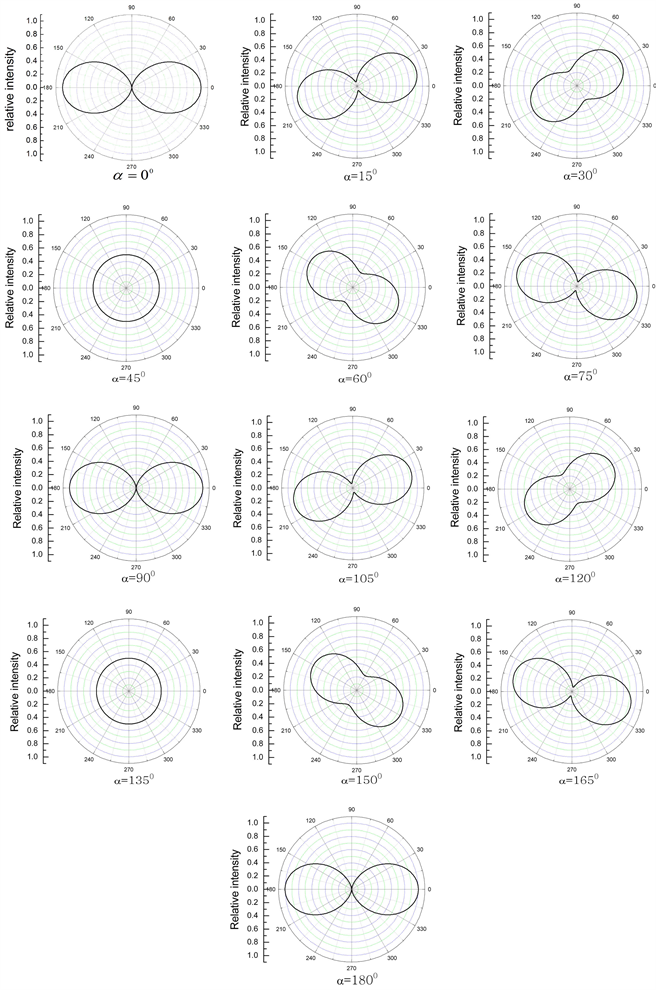Figure 3. For quarter-wave plate, the tracing curves of the emergent light intensity relative to the incident polarized light under different angle α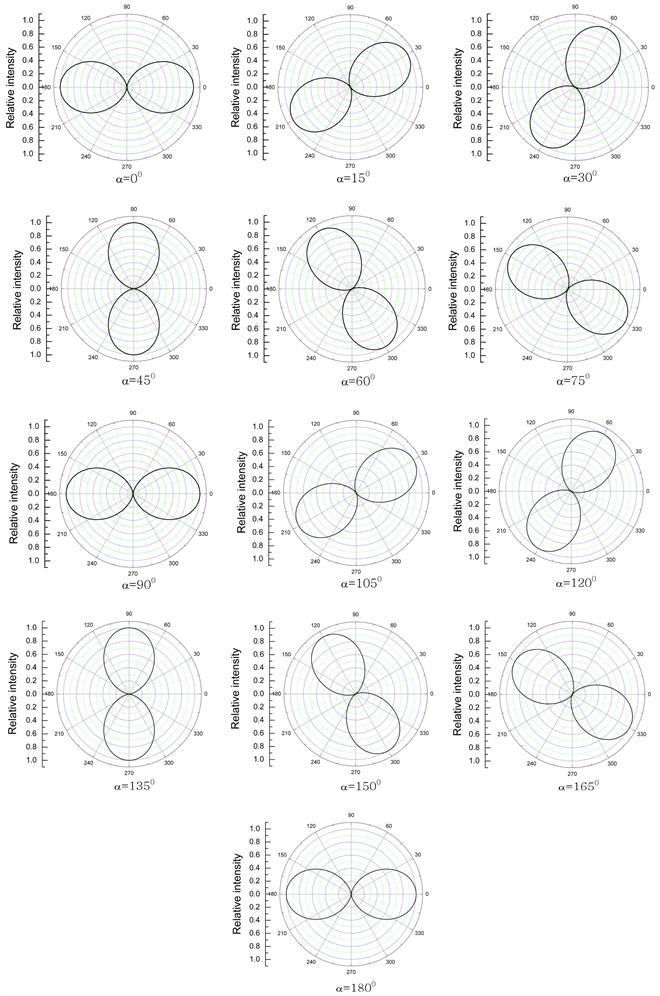Figure 4. For half-wave plate,the tracing curves of the emergent light intensity relative to the incident polarized light under different angle α

4. 实验装置及步骤

1) 实验装置：图5是实验装置图，左边是传统的电光实验装置，P1和P2分别为起偏器和检偏器，我们采用的BBO电光晶体半波电压1200 V。我们改进研制了偏光检测装置，其尺寸约10 × 6 × 4 cm，内置的检偏器(偏振片)转速为5转/秒，硅光电池探测，数字化后输入计算机，每半圈绘图一次画出实验曲线。

2) 实验步骤：首先调整光路与电光实验相同，当P1 ^ P2，调整到出现均匀对称的十字衍射条纹为止，然后接入旋转检偏系统，打开数据处理软件，实验准备工作就绪可进行旋转偏光实验。

5. 实验内容与实验结果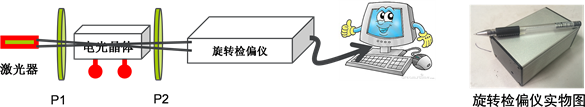Figure 5. Schematic diagram of the experiment set-upFigure 6. Experimental results of linearly polarized light: (a) Experimental curve; (b) Simulated curve; (c) Reductive result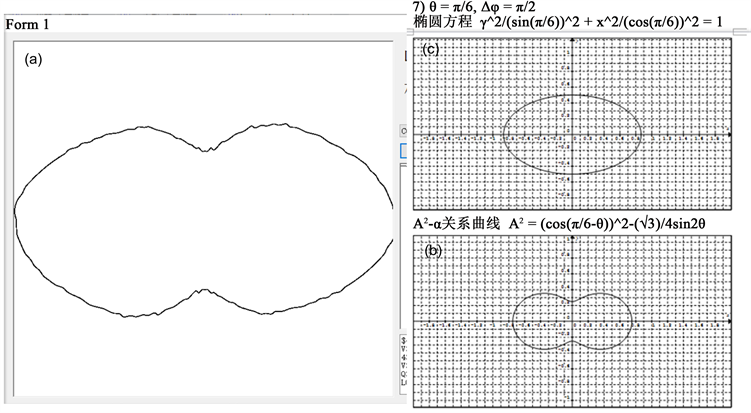Figure 7. Experimental results of elliptically polarized light: (a) Experimental curve; (b) Simulated curve; (c) Reductive result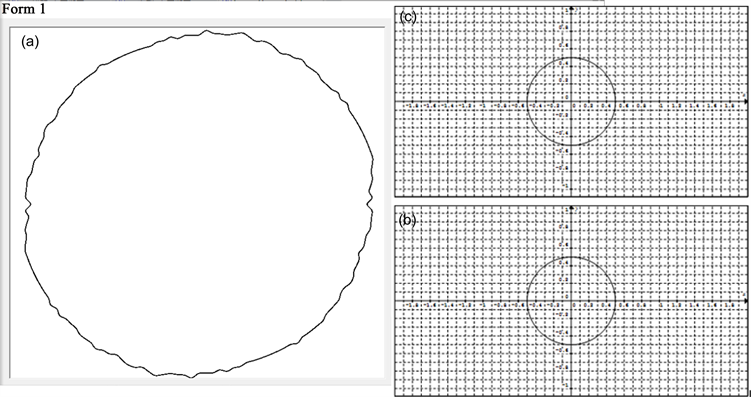Figure 8. Experimental results of circularly polarized light: (a) Experimental curve; (b) Simulated curve; (c) Reductive result

6. 结论

Theoretical Analysis of Rotating Polarization-Analyzer for Polarized Light and Its Application in Photoelectric Effect Experiment[J]. 现代物理, 2018, 08(01): 8-17. http://dx.doi.org/10.12677/MP.2018.81002

1. 1. 姚启钧. 光学教程[M]. 北京: 高等教育出版社, 1989.

2. 2. 程守洙, 江之永. 普通物理学第3册[M]. 北京: 高等教育出版社, 1989.

3. 3. 杨述武. 普通物理实验: 光学部分[M]. 北京: 高等教育出版社, 2000.

4. 4. 王秀芳, 陈世涛, 邱卫东. 偏振光试验方法的改进[J]. 大学物理实验, 2009, 22(1): 31-32.

5. 5. 王晓, 石顺祥, 马琳. 部分偏振光的表示方法[J]. 电子科技, 2009, 22(6): 78-80.

6. 6. 卢皓, 赵开春, 马强, 尤政. 采用连续旋转检偏器的天空偏振光探测装置设计与实现[J]. 宇航学报, 2014, 35(9): 1087-1094.

7. 7. 刘质斌, 丁剑平, 籍建葶, 张荣, 都有为. 傅里叶磁光谱仪中的起偏器和检偏器的自动定位及误差分析[J]. 中国激光, 2005, 32(5): 663-667.

8. 8. 朱化凤, 宋连科, 吴福全, 李国华, 左战春, 范树海. 泰勒检偏棱镜透射比随旋转角周期“抖动”的理论分析[J]. 中国激光, 2004, 31(1): 41-44.

9. 9. 龚奥博, 闫振, 沈志强, 吴理歌. 旋转射电暂现源研究进展[J]. 天文学进展, 2015, 33(4): 472-488.

10. 10. 蔡履中. 偏振光的杨氏干涉[J]. 大学物理, 2007, 26(6): 1-4.

11. 11. 上海交大物理实验中心组. 大学物理实验[M]. 上海: 上海交通大学出版社, 2015: 51.

12. 12. 陈皓, 史建新. 偏振光的理论分析与实验研究[J]. 沈阳师范大学学报, 2009, 27(4): 424-426.

13. 13. 王秀芳, 陈世涛, 邱卫东. 偏振光试验方法的改进[J]. 大学物理实验, 2009, 22(1): 31-32.

14. 14. 赵爽, 吴福全, 唐恒敬. 椭圆偏振光与部分偏振光检验的理论分析[J]. 激光技术, 2006, 30(4): 436-438.

15. 15. 何圣静. 物理学手册[M]. 北京: 机械工业出版社, 1989.

16. NOTES



*通讯作者。